Publicité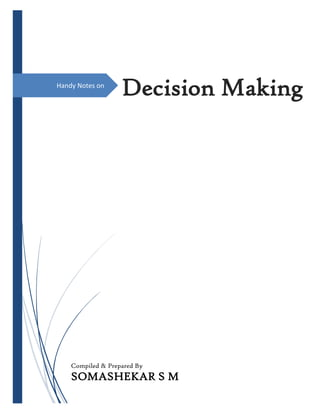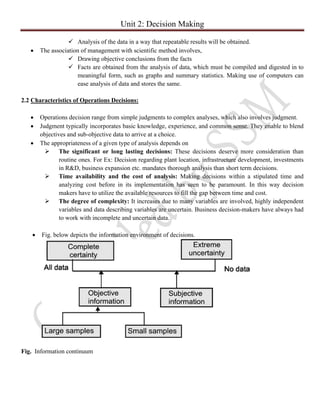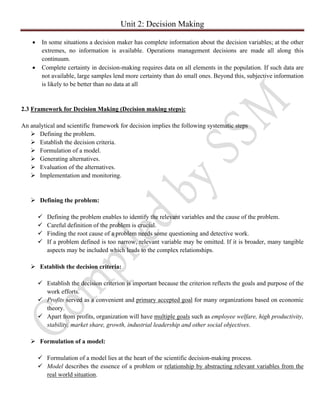Publicité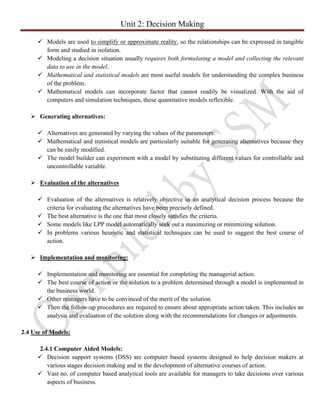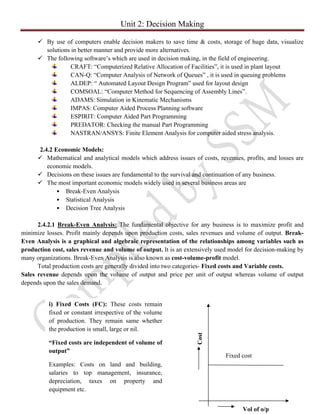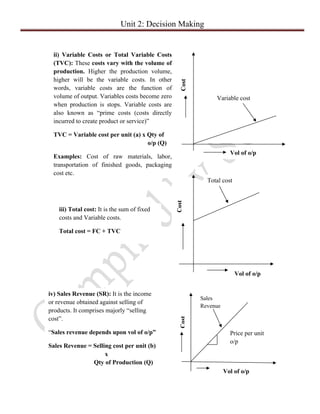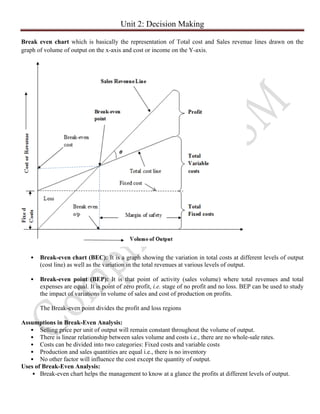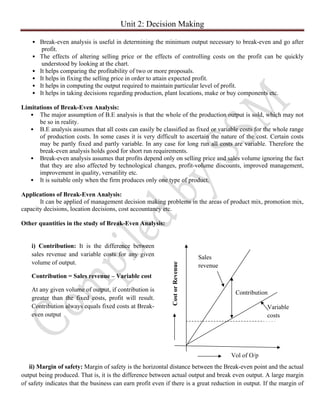Publicité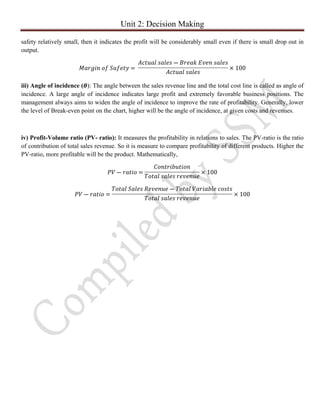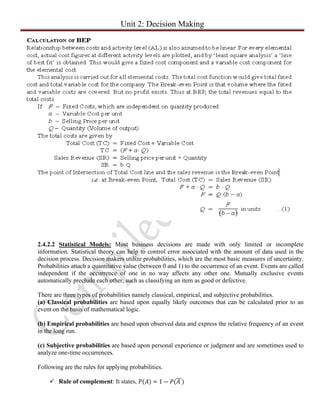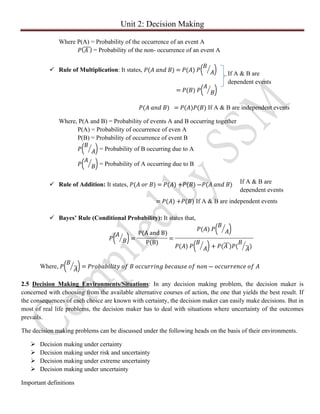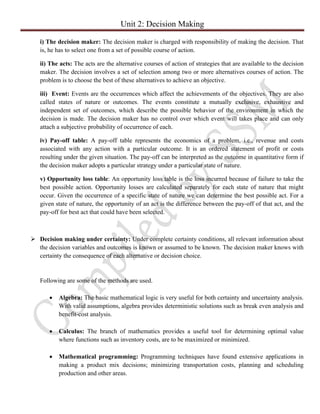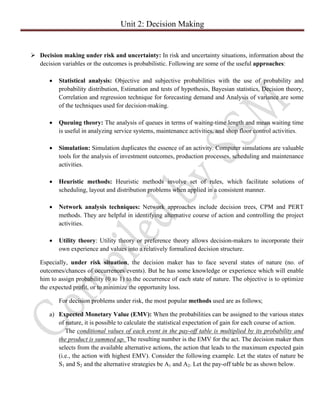Publicité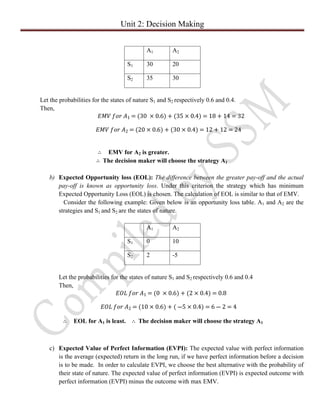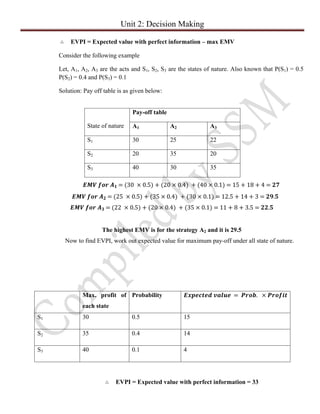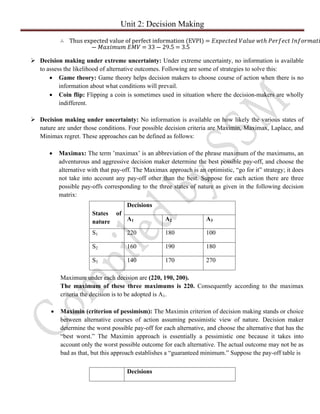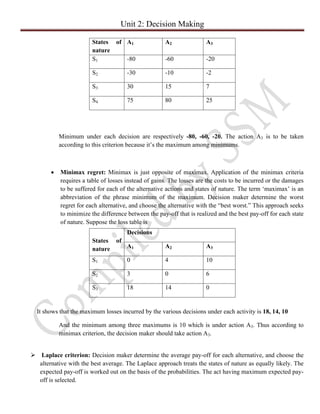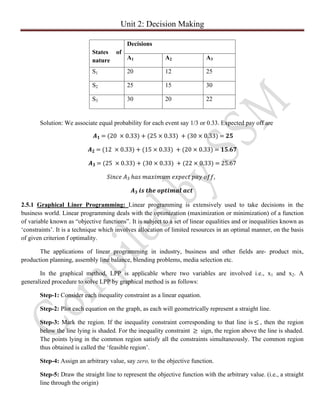Publicité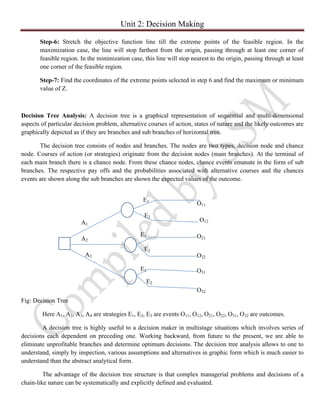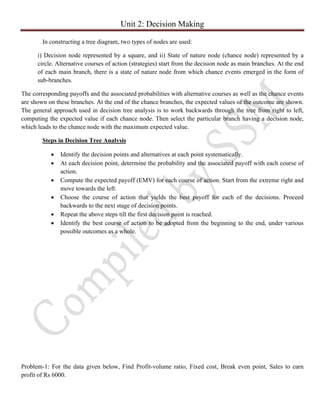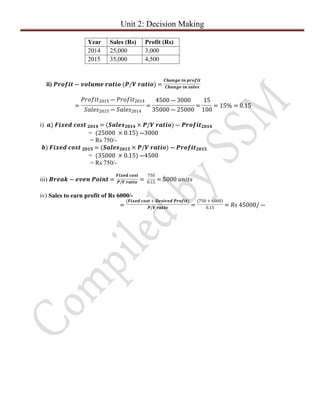Prochain SlideShare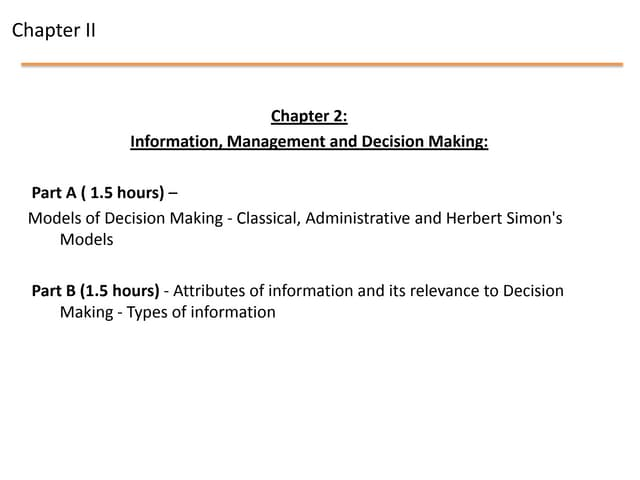Mis chapter 2 infomation, management and decision making
Chargement dans ... 3
1 sur 22
Publicité

### Decision making

1. Handy Notes on Decision Making Compiled & Prepared By SOMASHEKAR S M
2. Unit 2: Decision Making Contents  Introduction Definition  Characteristics of operations decisions,  Framework of decision making  Use of decision models,  Decision making environments,  Graphical linear programming,  Analysis and trade-offs. 2.0 Introduction: Thousands of business decisions are made every day. Not all the decisions will make or break the organization. But each one adds a measure of success or failure to the operations. Hence decision making essentially involves choosing a particular course of action, after considering the possible alternatives. 2.1 Definition: A decision is the conclusion of a process by which one chooses between two or more available alternative courses of action of the purpose attaining goals. The process is called decision making. According to Stephen P. Robbins, “decision making is defines as the selection of a preferred course of action from two or more alternatives.”  If the same process used at whole process of management then it is “Managerial decision making”.  Planning is the important managerial function which involves a series of decisions such as: What should do? When? How? Where? By when? By whom?  Even other functions of management such as organizing and controlling are also composed of decision making.  A decision is an act of choice wherein manger forms a conclusion about what must be done under a given situation.  Decision making involves two or more alternatives are in action selected to meet the requirements of the solution of a problem.  Decision making is an intellectual activity, because it calls for both judgment and imagination to select from among many alternatives.  Decision with regard to future course of action for the organization, over short or long terms. For example decisions taken in case of Inventory control Marketing 2.1.1 Scientific Decision Making:  Collaboration of SCIENCE with DECISION MAKING routes to ease and emphasize on improvement of decisions quality in a structured manner.  Scientific decision making rests upon  Knowledge of organization principles  Depends largely on empirical data
3. Unit 2: Decision Making  Analysis of the data in a way that repeatable results will be obtained.  The association of management with scientific method involves,  Drawing objective conclusions from the facts  Facts are obtained from the analysis of data, which must be compiled and digested in to meaningful form, such as graphs and summary statistics. Making use of computers can ease analysis of data and stores the same. 2.2 Characteristics of Operations Decisions:  Operations decision range from simple judgments to complex analyses, which also involves judgment.  Judgment typically incorporates basic knowledge, experience, and common sense. They enable to blend objectives and sub-objective data to arrive at a choice.  The appropriateness of a given type of analysis depends on  The significant or long lasting decisions: These decisions deserve more consideration than routine ones. For Ex: Decision regarding plant location, infrastructure development, investments in R&D, business expansion etc. mandates thorough analysis than short term decisions.  Time availability and the cost of analysis: Making decisions within a stipulated time and analyzing cost before in its implementation has seen to be paramount. In this way decision makers have to utilize the available resources to fill the gap between time and cost.  The degree of complexity: It increases due to many variables are involved, highly independent variables and data describing variables are uncertain. Business decision-makers have always had to work with incomplete and uncertain data.  Fig. below depicts the information environment of decisions. Fig. Information continuum
4. Unit 2: Decision Making  In some situations a decision maker has complete information about the decision variables; at the other extremes, no information is available. Operations management decisions are made all along this continuum.  Complete certainty in decision-making requires data on all elements in the population. If such data are not available, large samples lend more certainty than do small ones. Beyond this, subjective information is likely to be better than no data at all 2.3 Framework for Decision Making (Decision making steps): An analytical and scientific framework for decision implies the following systematic steps  Defining the problem.  Establish the decision criteria.  Formulation of a model.  Generating alternatives.  Evaluation of the alternatives.  Implementation and monitoring.  Defining the problem:  Defining the problem enables to identify the relevant variables and the cause of the problem.  Careful definition of the problem is crucial.  Finding the root cause of a problem needs some questioning and detective work.  If a problem defined is too narrow, relevant variable may be omitted. If it is broader, many tangible aspects may be included which leads to the complex relationships.  Establish the decision criteria:  Establish the decision criterion is important because the criterion reflects the goals and purpose of the work efforts.  Profits served as a convenient and primary accepted goal for many organizations based on economic theory.  Apart from profits, organization will have multiple goals such as employee welfare, high productivity, stability, market share, growth, industrial leadership and other social objectives.  Formulation of a model:  Formulation of a model lies at the heart of the scientific decision-making process.  Model describes the essence of a problem or relationship by abstracting relevant variables from the real world situation.
5. Unit 2: Decision Making  Models are used to simplify or approximate reality, so the relationships can be expressed in tangible form and studied in isolation.  Modeling a decision situation usually requires both formulating a model and collecting the relevant data to use in the model.  Mathematical and statistical models are most useful models for understanding the complex business of the problem.  Mathematical models can incorporate factor that cannot readily be visualized. With the aid of computers and simulation techniques, these quantitative models reflexible.  Generating alternatives:  Alternatives are generated by varying the values of the parameters.  Mathematical and statistical models are particularly suitable for generating alternatives because they can be easily modified.  The model builder can experiment with a model by substituting different values for controllable and uncontrollable variable.  Evaluation of the alternatives  Evaluation of the alternatives is relatively objective in an analytical decision process because the criteria for evaluating the alternatives have been precisely defined.  The best alternative is the one that most closely satisfies the criteria.  Some models like LPP model automatically seek out a maximizing or minimizing solution.  In problems various heuristic and statistical techniques can be used to suggest the best course of action.  Implementation and monitoring:  Implementation and monitoring are essential for completing the managerial action.  The best course of action or the solution to a problem determined through a model is implemented in the business world.  Other managers have to be convinced of the merit of the solution.  Then the follow-up procedures are required to ensure about appropriate action taken. This includes an analysis and evaluation of the solution along with the recommendations for changes or adjustments. 2.4 Use of Models: 2.4.1 Computer Aided Models:  Decision support systems (DSS) are computer based systems designed to help decision makers at various stages decision making and in the development of alternative courses of action.  Vast no. of computer based analytical tools are available for managers to take decisions over various aspects of business.
6. Unit 2: Decision Making  By use of computers enable decision makers to save time & costs, storage of huge data, visualize solutions in better manner and provide more alternatives.  The following software’s which are used in decision making, in the field of engineering. CRAFT: “Computerized Relative Allocation of Facilities”, it is used in plant layout CAN-Q: “Computer Analysis of Network of Queues” , it is used in queuing problems ALDEP: “ Automated Layout Design Program” used for layout design COMSOAL: “Computer Method for Sequencing of Assembly Lines”. ADAMS: Simulation in Kinematic Mechanisms IMPAS: Computer Aided Process Planning software ESPIRIT: Computer Aided Part Programming PREDATOR: Checking the manual Part Programming NASTRAN/ANSYS: Finite Element Analysis for computer aided stress analysis. 2.4.2 Economic Models:  Mathematical and analytical models which address issues of costs, revenues, profits, and losses are economic models.  Decisions on these issues are fundamental to the survival and continuation of any business.  The most important economic models widely used in several business areas are • Break-Even Analysis • Statistical Analysis • Decision Tree Analysis 2.4.2.1 Break-Even Analysis: The fundamental objective for any business is to maximize profit and minimize losses. Profit mainly depends upon production costs, sales revenues and volume of output. Break- Even Analysis is a graphical and algebraic representation of the relationships among variables such as production cost, sales revenue and volume of output. It is an extensively used model for decision-making by many organizations. Break-Even Analysis is also known as cost-volume-profit model. Total production costs are generally divided into two categories- Fixed costs and Variable costs. Sales revenue depends upon the volume of output and price per unit of output whereas volume of output depends upon the sales demand. i) Fixed Costs (FC): These costs remain fixed or constant irrespective of the volume of production. They remain same whether the production is small, large or nil. “Fixed costs are independent of volume of output” Examples: Costs on land and building, salaries to top management, insurance, depreciation, taxes on property and equipment etc. Cost Vol of o/p Fixed cost
7. Unit 2: Decision Making ii) Variable Costs or Total Variable Costs (TVC): These costs vary with the volume of production. Higher the production volume, higher will be the variable costs. In other words, variable costs are the function of volume of output. Variables costs become zero when production is stops. Variable costs are also known as “prime costs (costs directly incurred to create product or service)” TVC = Variable cost per unit (a) x Qty of o/p (Q) Examples: Cost of raw materials, labor, transportation of finished goods, packaging cost etc. Cost Vol of o/p iii) Total cost: It is the sum of fixed costs and Variable costs. Total cost = FC + TVC Variable cost Cost Vol of o/p Total cost iv) Sales Revenue (SR): It is the income or revenue obtained against selling of products. It comprises majorly “selling cost”. “Sales revenue depends upon vol of o/p” Sales Revenue = Selling cost per unit (b) x Qty of Production (Q) Cost Vol of o/p Sales Revenue Price per unit o/p
8. Unit 2: Decision Making Break even chart which is basically the representation of Total cost and Sales revenue lines drawn on the graph of volume of output on the x-axis and cost or income on the Y-axis. • Break-even chart (BEC): It is a graph showing the variation in total costs at different levels of output (cost line) as well as the variation in the total revenues at various levels of output. • Break-even point (BEP): It is that point of activity (sales volume) where total revenues and total expenses are equal. It is point of zero profit, i.e. stage of no profit and no loss. BEP can be used to study the impact of variations in volume of sales and cost of production on profits. The Break-even point divides the profit and loss regions Assumptions in Break-Even Analysis: • Selling price per unit of output will remain constant throughout the volume of output. • There is linear relationship between sales volume and costs i.e., there are no whole-sale rates. • Costs can be divided into two categories: Fixed costs and variable costs • Production and sales quantities are equal i.e., there is no inventory • No other factor will influence the cost except the quantity of output. Uses of Break-Even Analysis: • Break-even chart helps the management to know at a glance the profits at different levels of output.
9. Unit 2: Decision Making • Break-even analysis is useful in determining the minimum output necessary to break-even and go after profit. • The effects of altering selling price or the effects of controlling costs on the profit can be quickly understood by looking at the chart. • It helps comparing the profitability of two or more proposals. • It helps in fixing the selling price in order to attain expected profit. • It helps in computing the output required to maintain particular level of profit. • It helps in taking decisions regarding production, plant locations, make or buy components etc. Limitations of Break-Even Analysis: • The major assumption of B.E analysis is that the whole of the production output is sold, which may not be so in reality. • B.E analysis assumes that all costs can easily be classified as fixed or variable costs for the whole range of production costs. In some cases it is very difficult to ascertain the nature of the cost. Certain costs may be partly fixed and partly variable. In any case for long run all costs are variable. Therefore the break-even analysis holds good for short run requirements. • Break-even analysis assumes that profits depend only on selling price and sales volume ignoring the fact that they are also affected by technological changes, profit-volume discounts, improved management, improvement in quality, versatility etc. • It is suitable only when the firm produces only one type of product. Applications of Break-Even Analysis: It can be applied of management decision making problems in the areas of product mix, promotion mix, capacity decisions, location decisions, cost accountancy etc. Other quantities in the study of Break-Even Analysis: ii) Margin of safety: Margin of safety is the horizontal distance between the Break-even point and the actual output being produced. That is, it is the difference between actual output and break even output. A large margin of safety indicates that the business can earn profit even if there is a great reduction in output. If the margin of i) Contribution: It is the difference between sales revenue and variable costs for any given volume of output. Contribution = Sales revenue – Variable cost At any given volume of output, if contribution is greater than the fixed costs, profit will result. Contribution always equals fixed costs at Break- even output Vol of O/p CostorRevenue Contribution Sales revenue Variable costs
10. Unit 2: Decision Making safety relatively small, then it indicates the profit will be considerably small even if there is small drop out in output. 𝑀𝑎𝑟𝑔𝑖𝑛 𝑜𝑓 𝑆𝑎𝑓𝑒𝑡𝑦 = 𝐴𝑐𝑡𝑢𝑎𝑙 𝑠𝑎𝑙𝑒𝑠 ― 𝐵𝑟𝑒𝑎𝑘 𝐸𝑣𝑒𝑛 𝑠𝑎𝑙𝑒𝑠 𝐴𝑐𝑡𝑢𝑎𝑙 𝑠𝑎𝑙𝑒𝑠 × 100 iii) Angle of incidence ( ): The angle between the sales revenue line and the total cost line is called as angle of𝜽 incidence. A large angle of incidence indicates large profit and extremely favorable business positions. The management always aims to widen the angle of incidence to improve the rate of profitability. Generally, lower the level of Break-even point on the chart, higher will be the angle of incidence, at given costs and revenues. iv) Profit-Volume ratio (PV- ratio): It measures the profitability in relations to sales. The PV-ratio is the ratio of contribution of total sales revenue. So it is measure to compare profitability of different products. Higher the PV-ratio, more profitable will be the product. Mathematically, 𝑃𝑉 ― 𝑟𝑎𝑡𝑖𝑜 = 𝐶𝑜𝑛𝑡𝑟𝑖𝑏𝑢𝑡𝑖𝑜𝑛 𝑇𝑜𝑡𝑎𝑙 𝑠𝑎𝑙𝑒𝑠 𝑟𝑒𝑣𝑒𝑛𝑢𝑒 × 100 𝑃𝑉 ― 𝑟𝑎𝑡𝑖𝑜 = 𝑇𝑜𝑡𝑎𝑙 𝑆𝑎𝑙𝑒𝑠 𝑅𝑒𝑣𝑒𝑛𝑢𝑒 ― 𝑇𝑜𝑡𝑎𝑙 𝑉𝑎𝑟𝑖𝑎𝑏𝑙𝑒 𝑐𝑜𝑠𝑡𝑠 𝑇𝑜𝑡𝑎𝑙 𝑠𝑎𝑙𝑒𝑠 𝑟𝑒𝑣𝑒𝑛𝑢𝑒 × 100
11. Unit 2: Decision Making 2.4.2.2 Statistical Models: Most business decisions are made with only limited or incomplete information. Statistical theory can help to control error associated with the amount of data used in the decision process. Decision makers utilize probabilities, which are the most basic measures of uncertainty. Probabilities attach a quantitative value (between 0 and 1) to the occurrence of an event. Events are called independent if the occurrence of one in no way affects any other one. Mutually exclusive events automatically preclude each other, such as classifying an item as good or defective. There are three types of probabilities namely classical, empirical, and subjective probabilities. (a) Classical probabilities are based upon equally likely outcomes that can be calculated prior to an event on the basis of mathematical logic. (b) Empirical probabilities are based upon observed data and express the relative frequency of an event in the long run. (c) Subjective probabilities are based upon personal experience or judgment and are sometimes used to analyze one-time occurrences. Following are the rules for applying probabilities.  Rule of complement: It states, 𝑃(𝐴) = 1 ― 𝑃(𝐴 )
12. Unit 2: Decision Making Where P(A) = Probability of the occurrence of an event A = Probability of the non- occurrence of an event A𝑃( 𝐴 )  Rule of Multiplication: It states, 𝑃(𝐴 𝑎𝑛𝑑 𝐵) = 𝑃(𝐴) 𝑃( 𝐵 𝐴) = 𝑃(𝐵) 𝑃( 𝐴 𝐵) If A & B are independent events𝑃(𝐴 𝑎𝑛𝑑 𝐵) = 𝑃(𝐴)𝑃(𝐵) Where, P(A and B) = Probability of events A and B occurring together P(A) = Probability of occurrence of even A P(B) = Probability of occurrence of event B = Probability of B occurring due to A𝑃( 𝐵 𝐴) = Probability of A occurring due to B𝑃( 𝐴 𝐵)  Rule of Addition: It states, 𝑃(𝐴 𝑜𝑟 𝐵) = 𝑃(𝐴) +𝑃(𝐵) ―𝑃(𝐴 𝑎𝑛𝑑 𝐵) If A & B are independent events= 𝑃(𝐴) +𝑃(𝐵)  Bayes’ Rule (Conditional Probability): It states that, 𝑃( 𝐴 𝐵)= P(A and B) P(B) = 𝑃(𝐴) 𝑃( 𝐵 𝐴) 𝑃(𝐴) 𝑃( 𝐵 𝐴)+ 𝑃(𝐴 )𝑃( 𝐵 𝐴) Where, 𝑃( 𝐵 𝐴)= 𝑃𝑟𝑜𝑏𝑎𝑏𝑖𝑙𝑖𝑡𝑦 𝑜𝑓 𝐵 𝑜𝑐𝑐𝑢𝑟𝑟𝑖𝑛𝑔 𝑏𝑒𝑐𝑎𝑢𝑠𝑒 𝑜𝑓 𝑛𝑜𝑛 ― 𝑜𝑐𝑐𝑢𝑟𝑟𝑒𝑛𝑐𝑒 𝑜𝑓 𝐴 2.5 Decision Making Environments/Situations: In any decision making problem, the decision maker is concerned with choosing from the available alternative courses of action, the one that yields the best result. If the consequences of each choice are known with certainty, the decision maker can easily make decisions. But in most of real life problems, the decision maker has to deal with situations where uncertainty of the outcomes prevails. The decision making problems can be discussed under the following heads on the basis of their environments.  Decision making under certainty  Decision making under risk and uncertainty  Decision making under extreme uncertainty  Decision making under uncertainty Important definitions If A & B are dependent events If A & B are dependent events
13. Unit 2: Decision Making i) The decision maker: The decision maker is charged with responsibility of making the decision. That is, he has to select one from a set of possible course of action. ii) The acts: The acts are the alternative courses of action of strategies that are available to the decision maker. The decision involves a set of selection among two or more alternatives courses of action. The problem is to choose the best of these alternatives to achieve an objective. iii) Event: Events are the occurrences which affect the achievements of the objectives. They are also called states of nature or outcomes. The events constitute a mutually exclusive, exhaustive and independent set of outcomes, which describe the possible behavior of the environment in which the decision is made. The decision maker has no control over which event will takes place and can only attach a subjective probability of occurrence of each. iv) Pay-off table: A pay-off table represents the economics of a problem, i.e., revenue and costs associated with any action with a particular outcome. It is an ordered statement of profit or costs resulting under the given situation. The pay-off can be interpreted as the outcome in quantitative form if the decision maker adopts a particular strategy under a particular state of nature. v) Opportunity loss table: An opportunity loss table is the loss incurred because of failure to take the best possible action. Opportunity losses are calculated separately for each state of nature that might occur. Given the occurrence of a specific state of nature we can determine the best possible act. For a given state of nature, the opportunity of an act is the difference between the pay-off of that act, and the pay-off for best act that could have been selected.  Decision making under certainty: Under complete certainty conditions, all relevant information about the decision variables and outcomes is known or assumed to be known. The decision maker knows with certainty the consequence of each alternative or decision choice. Following are some of the methods are used.  Algebra: The basic mathematical logic is very useful for both certainty and uncertainty analysis. With valid assumptions, algebra provides deterministic solutions such as break even analysis and benefit-cost analysis.  Calculus: The branch of mathematics provides a useful tool for determining optimal value where functions such as inventory costs, are to be maximized or minimized.  Mathematical programming: Programming techniques have found extensive applications in making a product mix decisions; minimizing transportation costs, planning and scheduling production and other areas.
14. Unit 2: Decision Making  Decision making under risk and uncertainty: In risk and uncertainty situations, information about the decision variables or the outcomes is probabilistic. Following are some of the useful approaches:  Statistical analysis: Objective and subjective probabilities with the use of probability and probability distribution, Estimation and tests of hypothesis, Bayesian statistics, Decision theory, Correlation and regression technique for forecasting demand and Analysis of variance are some of the techniques used for decision-making.  Queuing theory: The analysis of queues in terms of waiting-time length and mean waiting time is useful in analyzing service systems, maintenance activities, and shop floor control activities.  Simulation: Simulation duplicates the essence of an activity. Computer simulations are valuable tools for the analysis of investment outcomes, production processes, scheduling and maintenance activities.  Heuristic methods: Heuristic methods involve set of rules, which facilitate solutions of scheduling, layout and distribution problems when applied in a consistent manner.  Network analysis techniques: Network approaches include decision trees, CPM and PERT methods. They are helpful in identifying alternative course of action and controlling the project activities.  Utility theory: Utility theory or preference theory allows decision-makers to incorporate their own experience and values into a relatively formalized decision structure. Especially, under risk situation, the decision maker has to face several states of nature (no. of outcomes/chances of occurrences/events). But he has some knowledge or experience which will enable him to assign probability (0 to 1) to the occurrence of each state of nature. The objective is to optimize the expected profit, or to minimize the opportunity loss. For decision problems under risk, the most popular methods used are as follows; a) Expected Monetary Value (EMV): When the probabilities can be assigned to the various states of nature, it is possible to calculate the statistical expectation of gain for each course of action. The conditional values of each event in the pay-off table is multiplied by its probability and the product is summed up. The resulting number is the EMV for the act. The decision maker then selects from the available alternative actions, the action that leads to the maximum expected gain (i.e., the action with highest EMV). Consider the following example. Let the states of nature be S1 and S2 and the alternative strategies be A1 and A2. Let the pay-off table be as shown below.
15. Unit 2: Decision Making A1 A2 S1 30 20 S2 35 30 Let the probabilities for the states of nature S1 and S2 respectively 0.6 and 0.4. Then, 𝐸𝑀𝑉 𝑓𝑜𝑟 𝐴1 = (30 × 0.6) + (35 × 0.4) = 18 + 14 = 32 𝐸𝑀𝑉 𝑓𝑜𝑟 𝐴2 = (20 × 0.6) + (30 × 0.4) = 12 + 12 = 24 EMV for A2 is greater.∴ The decision maker will choose the strategy A1∴ b) Expected Opportunity loss (EOL): The difference between the greater pay-off and the actual pay-off is known as opportunity loss. Under this criterion the strategy which has minimum Expected Opportunity Loss (EOL) is chosen. The calculation of EOL is similar to that of EMV. Consider the following example: Given below is an opportunity loss table. A1 and A2 are the strategies and S1 and S2 are the states of nature. A1 A2 S1 0 10 S2 2 -5 Let the probabilities for the states of nature S1 and S2 respectively 0.6 and 0.4 Then, 𝐸𝑂𝐿 𝑓𝑜𝑟 𝐴1 = (0 × 0.6) + (2 × 0.4) = 0.8 𝐸𝑂𝐿 𝑓𝑜𝑟 𝐴2 = (10 × 0.6) + ( ―5 × 0.4) = 6 ― 2 = 4 EOL for A1 is least. The decision maker will choose the strategy A1∴ ∴ c) Expected Value of Perfect Information (EVPI): The expected value with perfect information is the average (expected) return in the long run, if we have perfect information before a decision is to be made. In order to calculate EVPI, we choose the best alternative with the probability of their state of nature. The expected value of perfect information (EVPI) is expected outcome with perfect information (EVPI) minus the outcome with max EMV.
16. Unit 2: Decision Making EVPI = Expected value with perfect information – max EMV∴ Consider the following example Let, A1, A2, A3 are the acts and S1, S2, S3 are the states of nature. Also known that P(S1) = 0.5 P(S2) = 0.4 and P(S3) = 0.1 Solution: Pay off table is as given below: 𝑬𝑴𝑽 𝒇𝒐𝒓 𝑨 𝟏 = (30 × 0.5) + (20 × 0.4) + (40 × 0.1) = 15 + 18 + 4 = 𝟐𝟕 𝑬𝑴𝑽 𝒇𝒐𝒓 𝑨 𝟐 = (25 × 0.5) + (35 × 0.4) + (30 × 0.1) = 12.5 + 14 + 3 = 𝟐𝟗.𝟓 𝑬𝑴𝑽 𝒇𝒐𝒓 𝑨 𝟑 = (22 × 0.5) + (20 × 0.4) + (35 × 0.1) = 11 + 8 + 3.5 = 𝟐𝟐.𝟓 The highest EMV is for the strategy A2 and it is 29.5 Now to find EVPI, work out expected value for maximum pay-off under all state of nature. Max. profit of each state Probability 𝑬𝒙𝒑𝒆𝒄𝒕𝒆𝒅 𝒗𝒂𝒍𝒖𝒆 = 𝑷𝒓𝒐𝒃. × 𝑷𝒓𝒐𝒇𝒊𝒕 S1 30 0.5 15 S2 35 0.4 14 S3 40 0.1 4 EVPI = Expected value with perfect information = 33∴ Pay-off table State of nature A1 A2 A3 S1 30 25 22 S2 20 35 20 S3 40 30 35
17. Unit 2: Decision Making ∴ Thus expected value of perfect information (EVPI) = 𝐸𝑥𝑝𝑒𝑐𝑡𝑒𝑑 𝑉𝑎𝑙𝑢𝑒 𝑤𝑡ℎ 𝑃𝑒𝑟𝑓𝑒𝑐𝑡 𝐼𝑛𝑓𝑜𝑟𝑚𝑎𝑡𝑖 ― 𝑀𝑎𝑥𝑖𝑚𝑢𝑚 𝐸𝑀𝑉 = 33 ― 29.5 = 3.5  Decision making under extreme uncertainty: Under extreme uncertainty, no information is available to assess the likelihood of alternative outcomes. Following are some of strategies to solve this:  Game theory: Game theory helps decision makers to choose course of action when there is no information about what conditions will prevail.  Coin flip: Flipping a coin is sometimes used in situation where the decision-makers are wholly indifferent.  Decision making under uncertainty: No information is available on how likely the various states of nature are under those conditions. Four possible decision criteria are Maximin, Maximax, Laplace, and Minimax regret. These approaches can be defined as follows:  Maximax: The term ’maximax’ is an abbreviation of the phrase maximum of the maximums, an adventurous and aggressive decision maker determine the best possible pay-off, and choose the alternative with that pay-off. The Maximax approach is an optimistic, “go for it” strategy; it does not take into account any pay-off other than the best. Suppose for each action there are three possible pay-offs corresponding to the three states of nature as given in the following decision matrix: Maximum under each decision are (220, 190, 200). The maximum of these three maximums is 220. Consequently according to the maximax criteria the decision is to be adopted is A1.  Maximin (criterion of pessimism): The Maximin criterion of decision making stands or choice between alternative courses of action assuming pessimistic view of nature. Decision maker determine the worst possible pay-off for each alternative, and choose the alternative that has the “best worst.” The Maximin approach is essentially a pessimistic one because it takes into account only the worst possible outcome for each alternative. The actual outcome may not be as bad as that, but this approach establishes a “guaranteed minimum.” Suppose the pay-off table is Decisions States of nature A1 A2 A3 S1 220 180 100 S2 160 190 180 S3 140 170 270 Decisions
18. Unit 2: Decision Making Minimum under each decision are respectively -80, -60, -20. The action A3 is to be taken according to this criterion because it’s the maximum among minimums.  Minimax regret: Minimax is just opposite of maximax. Application of the minimax criteria requires a table of losses instead of gains. The losses are the costs to be incurred or the damages to be suffered for each of the alternative actions and states of nature. The term ‘maximax’ is an abbreviation of the phrase minimum of the maximum. Decision maker determine the worst regret for each alternative, and choose the alternative with the “best worst.” This approach seeks to minimize the difference between the pay-off that is realized and the best pay-off for each state of nature. Suppose the loss table is It shows that the maximum losses incurred by the various decisions under each activity is 18, 14, 10 And the minimum among three maximums is 10 which is under action A3. Thus according to minimax criterion, the decision maker should take action A3.  Laplace criterion: Decision maker determine the average pay-off for each alternative, and choose the alternative with the best average. The Laplace approach treats the states of nature as equally likely. The expected pay-off is worked out on the basis of the probabilities. The act having maximum expected pay- off is selected. States of nature A1 A2 A3 S1 -80 -60 -20 S2 -30 -10 -2 S3 30 15 7 S4 75 80 25 Decisions States of nature A1 A2 A3 S1 0 4 10 S2 3 0 6 S3 18 14 0
19. Unit 2: Decision Making Solution: We associate equal probability for each event say 1/3 or 0.33. Expected pay off are 𝑨 𝟏 = (20 × 0.33) + (25 × 0.33) + (30 × 0.33) = 𝟐𝟓 𝑨 𝟐 = (12 × 0.33) + (15 × 0.33) + (20 × 0.33) = 𝟏𝟓.𝟔𝟕 𝑨 𝟑 = (25 × 0.33) + (30 × 0.33) + (22 × 0.33) = 25.67 𝑆𝑖𝑛𝑐𝑒 𝐴3 ℎ𝑎𝑠 𝑚𝑎𝑥𝑖𝑚𝑢𝑚 𝑒𝑥𝑝𝑒𝑐𝑡 𝑝𝑎𝑦 𝑜𝑓𝑓, 𝑨 𝟑 𝒊𝒔 𝒕𝒉𝒆 𝒐𝒑𝒕𝒊𝒎𝒂𝒍 𝒂𝒄𝒕 2.5.1 Graphical Liner Programming: Linear programming is extensively used to take decisions in the business world. Linear programming deals with the optimization (maximization or minimization) of a function of variable known as “objective functions”. It is subject to a set of linear equalities and or inequalities known as ‘constraints’. It is a technique which involves allocation of limited resources in an optimal manner, on the basis of given criterion f optimality. The applications of linear programming in industry, business and other fields are- product mix, production planning, assembly line balance, blending problems, media selection etc. In the graphical method, LPP is applicable where two variables are involved i.e., x1 and x2. A generalized procedure to solve LPP by graphical method is as follows: Step-1: Consider each inequality constraint as a linear equation. Step-2: Plot each equation on the graph, as each will geometrically represent a straight line. Step-3: Mark the region. If the inequality constraint corresponding to that line is , then the region≤ below the line lying is shaded. For the inequality constraint sign, the region above the line is shaded.≥ The points lying in the common region satisfy all the constraints simultaneously. The common region thus obtained is called the ‘feasible region’. Step-4: Assign an arbitrary value, say zero, to the objective function. Step-5: Draw the straight line to represent the objective function with the arbitrary value. (i.e., a straight line through the origin) Decisions States of nature A1 A2 A3 S1 20 12 25 S2 25 15 30 S3 30 20 22
20. Unit 2: Decision Making Step-6: Stretch the objective function line till the extreme points of the feasible region. In the maximization case, the line will stop farthest from the origin, passing through at least one corner of feasible region. In the minimization case, this line will stop nearest to the origin, passing through at least one corner of the feasible region. Step-7: Find the coordinates of the extreme points selected in step 6 and find the maximum or minimum value of Z. Decision Tree Analysis: A decision tree is a graphical representation of sequential and multi-dimensional aspects of particular decision problem, alternative courses of action, states of nature and the likely outcomes are graphically depicted as if they are branches and sub branches of horizontal tree. The decision tree consists of nodes and branches. The nodes are two types, decision node and chance node. Courses of action (or strategies) originate from the decision nodes (main branches). At the terminal of each main branch there is a chance node. From these chance nodes, chance events emanate in the form of sub branches. The respective pay offs and the probabilities associated with alternative courses and the chances events are shown along the sub branches are shown the expected values of the outcome. Fig: Decision Tree Here A1, A2, A3, A4 are strategies E1, E2, E3 are events O11, O12, O21, O22, O31, O32 are outcomes. A decision tree is highly useful to a decision maker in multistage situations which involves series of decisions each dependent on preceding one. Working backward, from future to the present, we are able to eliminate unprofitable branches and determine optimum decisions. The decision tree analysis allows to one to understand, simply by inspection, various assumptions and alternatives in graphic form which is much easier to understand than the abstract analytical form. The advantage of the decision tree structure is that complex managerial problems and decisions of a chain-like nature can be systematically and explicitly defined and evaluated. A1 A2 A3 E1 E2 E1 E2 E1 E2 O11 O21 O12 O31 O22 O32
21. Unit 2: Decision Making In constructing a tree diagram, two types of nodes are used: i) Decision node represented by a square, and ii) State of nature node (chance node) represented by a circle. Alternative courses of action (strategies) start from the decision node as main branches. At the end of each main branch, there is a state of nature node from which chance events emerged in the form of sub-branches. The corresponding payoffs and the associated probabilities with alternative courses as well as the chance events are shown on these branches. At the end of the chance branches, the expected values of the outcome are shown. The general approach used in decision tree analysis is to work backwards through the tree from right to left, computing the expected value if each chance node. Then select the particular branch having a decision node, which leads to the chance node with the maximum expected value. Steps in Decision Tree Analysis  Identify the decision points and alternatives at each point systematically.  At each decision point, determine the probability and the associated payoff with each course of action.  Compute the expected payoff (EMV) for each course of action. Start from the extreme right and move towards the left.  Choose the course of action that yields the best payoff for each of the decisions. Proceed backwards to the next stage of decision points.  Repeat the above steps till the first decision point is reached.  Identify the best course of action to be adopted from the beginning to the end, under various possible outcomes as a whole. Problem-1: For the data given below, Find Profit-volume ratio, Fixed cost, Break even point, Sales to earn profit of Rs 6000.
22. Unit 2: Decision Making Year Sales (Rs) Profit (Rs) 2014 25,000 3,000 2015 35,000 4,500 ii) 𝑷𝒓𝒐𝒇𝒊𝒕 ― 𝒗𝒐𝒍𝒖𝒎𝒆 𝒓𝒂𝒕𝒊𝒐 (𝑷/𝑽 𝒓𝒂𝒕𝒊𝒐) = 𝑪𝒉𝒂𝒏𝒈𝒆 𝒊𝒏 𝒑𝒓𝒐𝒇𝒊𝒕 𝑪𝒉𝒂𝒏𝒈𝒆 𝒊𝒏 𝒔𝒂𝒍𝒆𝒔 = 𝑃𝑟𝑜𝑓𝑖𝑡2015 ― 𝑃𝑟𝑜𝑓𝑖𝑡2014 𝑆𝑎𝑙𝑒𝑠2015 ― 𝑆𝑎𝑙𝑒𝑠2014 = 4500 ― 3000 35000 ― 25000 = 15 100 = 15% = 0.15 i) 𝒂) 𝑭𝒊𝒙𝒆𝒅 𝒄𝒐𝒔𝒕 𝟐𝟎𝟏𝟒 = (𝑺𝒂𝒍𝒆𝒔 𝟐𝟎𝟏𝟒 × 𝑷/𝑽 𝒓𝒂𝒕𝒊𝒐) ― 𝑷𝒓𝒐𝒇𝒊𝒕 𝟐𝟎𝟏𝟒 = (25000 × 0.15) ―3000 = Rs 750/- 𝒃) 𝑭𝒊𝒙𝒆𝒅 𝒄𝒐𝒔𝒕 𝟐𝟎𝟏𝟓 = (𝑺𝒂𝒍𝒆𝒔 𝟐𝟎𝟏𝟓 × 𝑷/𝑽 𝒓𝒂𝒕𝒊𝒐) ― 𝑷𝒓𝒐𝒇𝒊𝒕 𝟐𝟎𝟏𝟓 = (35000 × 0.15) ―4500 = Rs 750/- iii) 𝑩𝒓𝒆𝒂𝒌 ― 𝒆𝒗𝒆𝒏 𝑷𝒐𝒊𝒏𝒕 = 𝑭𝒊𝒙𝒆𝒅 𝒄𝒐𝒔𝒕 𝑷/𝑽 𝒓𝒂𝒕𝒊𝒐 = 750 0.15 = 5000 𝑢𝑛𝑖𝑡𝑠 iv) Sales to earn profit of Rs 6000/- = (𝑭𝒊𝒙𝒆𝒅 𝒄𝒐𝒔𝒕 + 𝑫𝒆𝒔𝒊𝒓𝒆𝒅 𝑷𝒓𝒐𝒇𝒊𝒕) 𝑷/𝑽 𝒓𝒂𝒕𝒊𝒐 = (750 + 6000) 0.15 = 𝑅𝑠 45000/ ―
Publicité